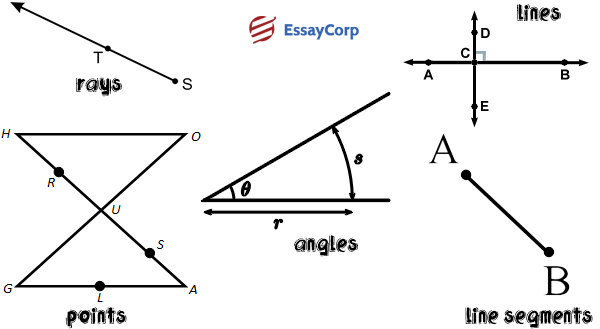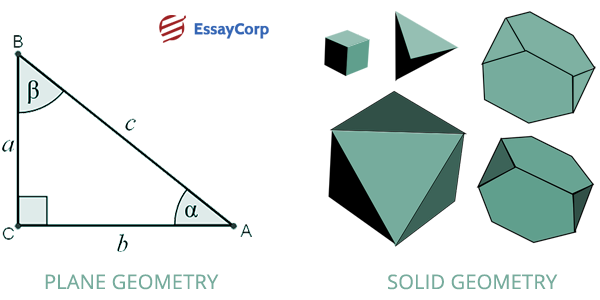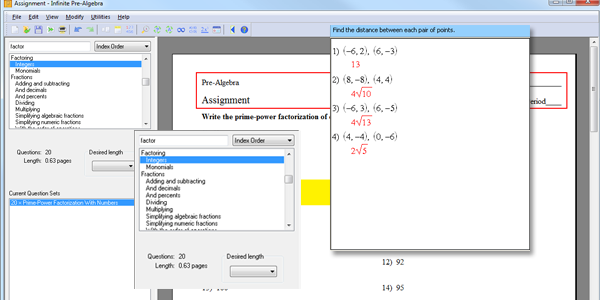The term geometry implies the measurement of earth. The creation of geometrical figures and the examination of their properties is what we learn from geometrical studies. It is that branch of mathematics which studies the shapes, lines, properties, angles etc. A mathematician who studies the geometrics is called a geometer. Geometry enables a person to predict about the physical world through an intellectual process. It handles two dimensional, three dimensional and flat shapes. Basic geometric shapes such as line segments, lines, points, rays etc. create the two dimensional, three dimensional and flat shapes.

## Euclid Of Alexandria

Alexandria was a mathematician from Greece. He is also known as the father of geometry.  He was also considered as the most outstanding mathematicians. During the reign of Ptolemy he used to teach mathematics. He wrote a book about geometry namely ‘The Elements’ which was a huge success. It became the most famous book about the systematic discussion of geometry. The book contained rules of geometry.

## Some Of The Important Concepts Of Geometry Are:-Important Concepts Of Geometry

### Points

– A point works as a location in geometry. The point is explained by a dot. A point has no length, size or depth. It is the most significant and the most basic object in geometry. Not only in geometry, a point has an equal significance in our regular life as well, for instance, it shows our location on a digital map.

### Lines

– A line in geometry is a bar that keeps on going. It is as basic as a point. A line has one dimension which is its length. A line is something that can always be found around us. Example- on the doors, ceilings, televisions, beds or almost in everything that can be seen.

### Line Segments

– In geometry the line segment is a part of a line. It has arrows in its each ending point. A line keeps on going whereas a line segment has a specific start and end. It basically is a part of a line with two end points. Example- a table edge, a book’s spine etc.

### Rays

– A ray is a line that starts from a point and keeps on going to the infinity. It is the consolidation of a line and a line segment. It has a terminating and extending end. Examples of rays that we find in our daily life are, rays from sun, torches, projectors etc.

### Angles

– An angle is a figure formed by two lines that go to different directions but their starting point is the same. Some features of an angle are Legs, Vertexes, Interiors and Exteriors. There are four types of angles, right angle ex- a square container, obtuse angle ex- door hinges, acute angle ex- spokes of a bike and straight angle ex- edge of a paper or a table.

## Parallel Lines And Perpendicular Lines

Parallel lines are two different lines that cannot have a common point. The parallel lines can never touch and they are always at the same distance apart from each other. They should be drawn on the same plane. To understand the connection between various sides of shapes and paths of objects, parallel lines are useful. Two lines that are intersecting each other make an angle of 90 degrees and they are called perpendicular lines. We can find perpendicular lines everywhere in the world and not only in graphs and sheets like crossing patterns on roads. Two lines intersect each other making the angle a right angle is known as perpendicular lines.

## What Is Plane And Solid Geometry?The Two Broad Types Of Geometry

The Plane and Solid geometry are basically the two broad types of geometry.

### Plane Geometry

– Plane geometry talks about the objects that are flat in nature. All the shapes in plane geometry appear in flat shapes.  A plane has no thickness and it continues to go on in both directions. It studies shapes that can be drawn on a paper like, triangle, square, circle etc. which is also called the 2d shapes. It is a little difficult to find the plane geometry examples in our regular life but we can take a piece of paper, surface of wall as examples.

### Solid Geometry

– Solid geometry studies the three dimensional shapes. The three dimensions include thickness, length and breadth. Some examples of three dimensional shapes are cubes, cylinders, cones etc. These are easy to find in our daily life.

## Geometry Assignment Kuta SoftwareUseful Math Tools Provided By Kuta Software

Based in Rockville Maryland, the Kuta software is a small company that provides useful math tools to the maths teachers. The Kuta software provides the teachers some products that help in creating some great math problems that they want to give their students in class for practice. It lets the teacher to teach in a creative way to their students which makes the learning interesting.

Geometry is a subject that we study in academic life, and deal in real life. So, it is necessary to teach students through everyday examples practically. Practical geometry lessons help the students to understand the nitty-gritties of its concepts.

Author:
Category :
Publish Date :
Social Sharing